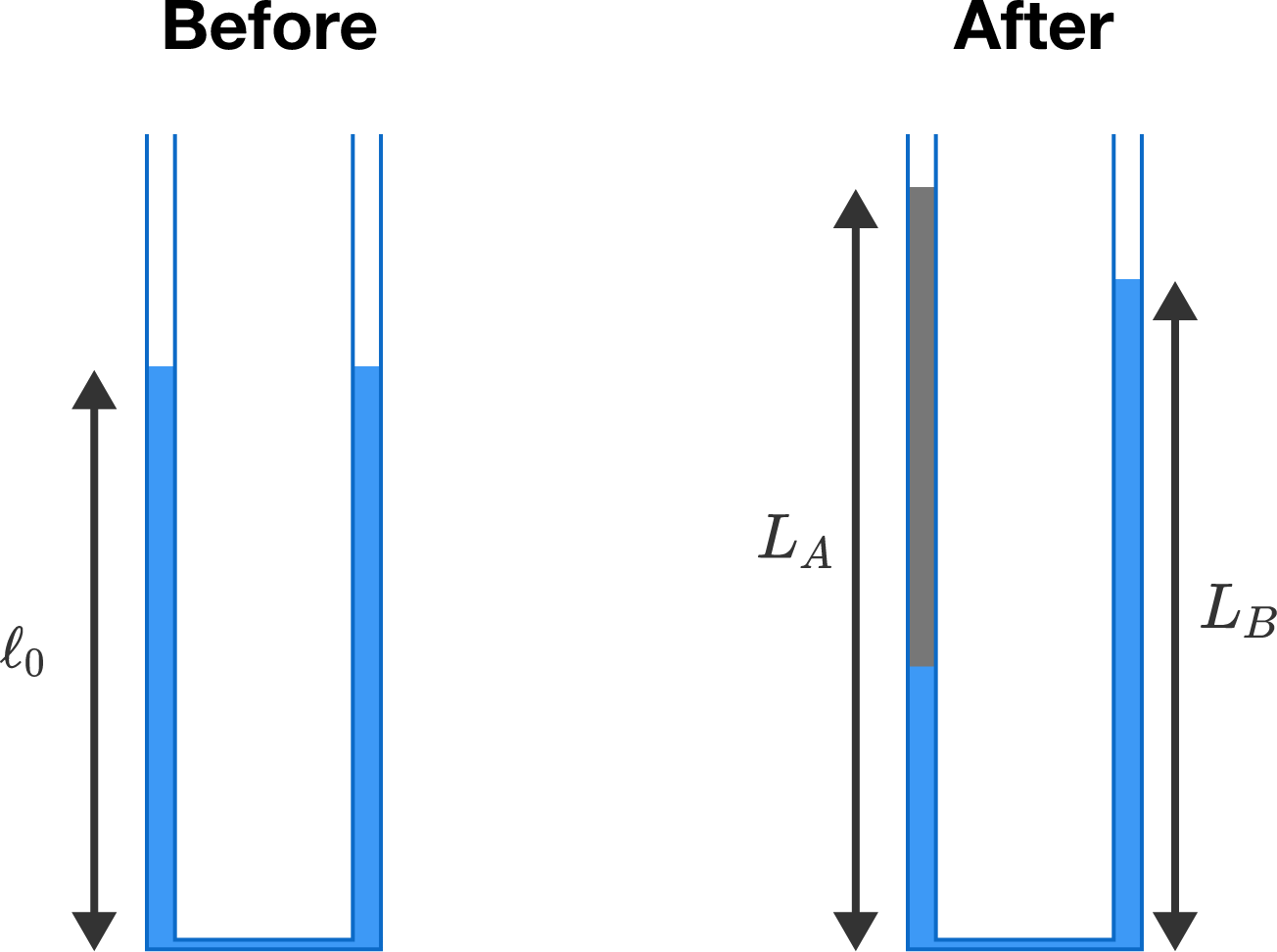# U-tubeInitially, the U-tube shown above is filled with water, and the level on each side is $\ell_0 = \SI{5}{\centi\meter}.$ After some oil is poured on side $A,$ the level on that side becomes $L_A = \SI{7.3}{\centi\meter}.$

What is $L_B$ $($in $\si{\centi\meter})?$

Assume that the water and oil do not mix, and that their densities are related by $\rho_\textrm{oil} = 0.85\rho_\textrm{water}.$

×